Test: Linear Equations in Two Variables- Case Based Type Questions- 2

# Test: Linear Equations in Two Variables- Case Based Type Questions- 2 - EmSAT Achieve

Test Description

## 10 Questions MCQ Test Mathematics for EmSAT Achieve - Test: Linear Equations in Two Variables- Case Based Type Questions- 2

Test: Linear Equations in Two Variables- Case Based Type Questions- 2 for EmSAT Achieve 2023 is part of Mathematics for EmSAT Achieve preparation. The Test: Linear Equations in Two Variables- Case Based Type Questions- 2 questions and answers have been prepared according to the EmSAT Achieve exam syllabus.The Test: Linear Equations in Two Variables- Case Based Type Questions- 2 MCQs are made for EmSAT Achieve 2023 Exam. Find important definitions, questions, notes, meanings, examples, exercises, MCQs and online tests for Test: Linear Equations in Two Variables- Case Based Type Questions- 2 below.
Solutions of Test: Linear Equations in Two Variables- Case Based Type Questions- 2 questions in English are available as part of our Mathematics for EmSAT Achieve for EmSAT Achieve & Test: Linear Equations in Two Variables- Case Based Type Questions- 2 solutions in Hindi for Mathematics for EmSAT Achieve course. Download more important topics, notes, lectures and mock test series for EmSAT Achieve Exam by signing up for free. Attempt Test: Linear Equations in Two Variables- Case Based Type Questions- 2 | 10 questions in 20 minutes | Mock test for EmSAT Achieve preparation | Free important questions MCQ to study Mathematics for EmSAT Achieve for EmSAT Achieve Exam | Download free PDF with solutions
 1 Crore+ students have signed up on EduRev. Have you?
Test: Linear Equations in Two Variables- Case Based Type Questions- 2 - Question 1

### The force applied on a body is directly proportional to the acceleration produced in the body. Assume x be acceleration produced in the body and y be the force. Take constant as 10.(S.I unit of force is Newton and S.I. unit of acceleration is m/sec2.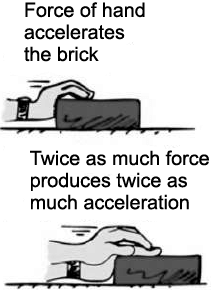Q. Name the type of equation formed in part(A).

Detailed Solution for Test: Linear Equations in Two Variables- Case Based Type Questions- 2 - Question 1
y = cx is a linear equation as highest power of x is 1.
Test: Linear Equations in Two Variables- Case Based Type Questions- 2 - Question 2

### The force applied on a body is directly proportional to the acceleration produced in the body. Assume x be acceleration produced in the body and y be the force. Take constant as 10.(S.I unit of force is Newton and S.I. unit of acceleration is m/sec2.Q. Find acceleration produced in the body, if force applied is 10 newtons.

Detailed Solution for Test: Linear Equations in Two Variables- Case Based Type Questions- 2 - Question 2
Given,

Force applied = 10 newtons

c = 10

Since, y = cx

⇒ 10 = 10x

⇒ x = 1

Thus, acceleration produced = 1 m/sec2

Test: Linear Equations in Two Variables- Case Based Type Questions- 2 - Question 3

### The force applied on a body is directly proportional to the acceleration produced in the body. Assume x be acceleration produced in the body and y be the force. Take constant as 10.(S.I unit of force is Newton and S.I. unit of acceleration is m/sec2.Q. Write an equation to express the above situation.

Detailed Solution for Test: Linear Equations in Two Variables- Case Based Type Questions- 2 - Question 3
y is directly proportional to x.

⇒ y = cx where c is a constant.

Test: Linear Equations in Two Variables- Case Based Type Questions- 2 - Question 4

The force applied on a body is directly proportional to the acceleration produced in the body. Assume x be acceleration produced in the body and y be the force. Take constant as 10.(S.I unit of force is Newton and S.I. unit of acceleration is m/sec2.Q. Equation formed on above situation has ______ solution(s).

Detailed Solution for Test: Linear Equations in Two Variables- Case Based Type Questions- 2 - Question 4
A linear equations has infinitely many solutions.
Test: Linear Equations in Two Variables- Case Based Type Questions- 2 - Question 5

The force applied on a body is directly proportional to the acceleration produced in the body. Assume x be acceleration produced in the body and y be the force. Take constant as 10.(S.I unit of force is Newton and S.I. unit of acceleration is m/sec2.Q. Find force applied, if acceleration produced is 2.5m/sec2.

Detailed Solution for Test: Linear Equations in Two Variables- Case Based Type Questions- 2 - Question 5
Given,

acceleration produced = 2.5 m/sec2

y = cx,

y = 10 × 2.5

= 25 newtons

Test: Linear Equations in Two Variables- Case Based Type Questions- 2 - Question 6

Temperature is a measure of the warmth or coldness of an object or substance with reference to some standard value.

In countries like USA and Canada, temperature is measured in Fahrenheit, whereas in countries like India, it is measured in Celsius.

An equation that converts Fahrenheit to Celsius is: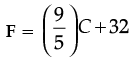On reading the above information, some queries come in Aman’s mind. Help him to resolve his following queries:

Q. How many variables are there in the equation,Detailed Solution for Test: Linear Equations in Two Variables- Case Based Type Questions- 2 - Question 6
There are two variables, C and F.
Test: Linear Equations in Two Variables- Case Based Type Questions- 2 - Question 7

Temperature is a measure of the warmth or coldness of an object or substance with reference to some standard value.

In countries like USA and Canada, temperature is measured in Fahrenheit, whereas in countries like India, it is measured in Celsius.

An equation that converts Fahrenheit to Celsius is:On reading the above information, some queries come in Aman’s mind. Help him to resolve his following queries:

Q. If the temperature is 95°F, what is the temperature in Celsius ?

Detailed Solution for Test: Linear Equations in Two Variables- Case Based Type Questions- 2 - Question 7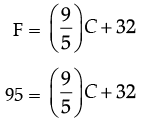95 × 5 = 9C + 160

475 = 9C + 160

9C = 475 – 160

9C = 315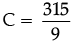C = 35°

Test: Linear Equations in Two Variables- Case Based Type Questions- 2 - Question 8

Temperature is a measure of the warmth or coldness of an object or substance with reference to some standard value.

In countries like USA and Canada, temperature is measured in Fahrenheit, whereas in countries like India, it is measured in Celsius.

An equation that converts Fahrenheit to Celsius is:On reading the above information, some queries come in Aman’s mind. Help him to resolve his following queries:

Q. What is the type of equation, F =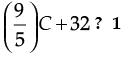Detailed Solution for Test: Linear Equations in Two Variables- Case Based Type Questions- 2 - Question 8
As its highest power is 2. Thus, it is a linear equation.
Test: Linear Equations in Two Variables- Case Based Type Questions- 2 - Question 9

Temperature is a measure of the warmth or coldness of an object or substance with reference to some standard value.

In countries like USA and Canada, temperature is measured in Fahrenheit, whereas in countries like India, it is measured in Celsius.

An equation that converts Fahrenheit to Celsius is:On reading the above information, some queries come in Aman’s mind. Help him to resolve his following queries:

Q. If the temperature is 30°C, what is the temperature in Fahrenheit ?

Detailed Solution for Test: Linear Equations in Two Variables- Case Based Type Questions- 2 - Question 9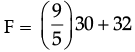= 54 + 32

= 86

Test: Linear Equations in Two Variables- Case Based Type Questions- 2 - Question 10

Temperature is a measure of the warmth or coldness of an object or substance with reference to some standard value.

In countries like USA and Canada, temperature is measured in Fahrenheit, whereas in countries like India, it is measured in Celsius.

An equation that converts Fahrenheit to Celsius is:On reading the above information, some queries come in Aman’s mind. Help him to resolve his following queries:

Q. What is a temperature which is numerically the same in both Fahrenheit and Celsius ?

Detailed Solution for Test: Linear Equations in Two Variables- Case Based Type Questions- 2 - Question 10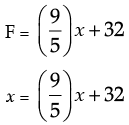5x = 9x + 160

5x – 9x = 160

– 4x = 160

x = – 40

## Mathematics for EmSAT Achieve

151 videos|237 docs|254 tests
Information about Test: Linear Equations in Two Variables- Case Based Type Questions- 2 Page
In this test you can find the Exam questions for Test: Linear Equations in Two Variables- Case Based Type Questions- 2 solved & explained in the simplest way possible. Besides giving Questions and answers for Test: Linear Equations in Two Variables- Case Based Type Questions- 2, EduRev gives you an ample number of Online tests for practice

## Mathematics for EmSAT Achieve

151 videos|237 docs|254 tests